# MCQs on Electric Circuits

##### Page 49 of 63. Go to page 1 2 3 4 5 6 7 8 9 10 11 12 13 14 15 16 17 18 19 20 21 22 23 24 25 26 27 28 29 30 31 32 33 34 35 36 37 38 39 40 41 42 43 44 45 46 47 48 49 50 51 52 53 54 55 56 57 58 59 60 61 62 63
01․ When 125 V is applied across a 250 V, 100 W filament incandescent bulbs, the power consumption is
25 W.
12.5 W.
50 W.
100 W.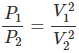02․ Three resistors of 4 ohm, 6 ohm and 8 ohm are in parallel. In which resistor, power dissipation will be maximum
8 Ohm.
4 Ohm.
6 Ohm.
equal in all resistors.

In parallel connection,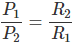03․ The circuit whose properties are same in either direction is known as
reversible circuit.
bilateral circuit.
unilateral circuit.
passive circuit .

A bilateral circuit is a circuit whose properties are same in either direction.

04․ Kirchhoff’s voltage law is used for
nodal analysis.
finding equivalent resistance.
representing a current source.
loop analysis.

KVL states that the sum of voltage drops is equal to sum of voltage rise in a closed loop.

05․ Kirchhoff’s current law is used for
nodal analysis.
loop analysis.
finding equivalent resistance.
representing a current source.

KCL states that the sum of currents entering a node is equal to sum of currents leaving the node.

06․ For Norton’s equivalent circuit
Isc = Voc / Req.
Isc = Voc Req.
Voc = Isc / Req.
Voc = Req /Isc.

The Norton Equivalent circuit is represented by a current source and equivalent Norton resistance in parallel. The value of the current source is the short circuit current through load terminals.

07․ Flux in a magnetic circuit can be compared in an electric circuit to
voltage.
current.
resistance.
inductance.

Current in electric circuit is analogous to flux in magnetic circuit. In an electric circuit, EMF is necessary to cause the flow of current; similarly magneto-motive force is required to create the magnetic flux in the magnetic circuit. Also, as the current flowing in an electric circuit depends upon the EMF and resistance of the circuit, similarly the magnetic flux or number of lines of force produced in any magnetic circuit depends upon the magneto-motive force acting in the circuit and reactance of the circuit.

08․ If the peak value of a sine wave is 100 V, then its RMS value will be
88 V.
70.7 V.
63.6 V.
100 V.

RMS value is 0.707 times the peak value for sinusoidal signals. The RMS value of any signal u(t) is given by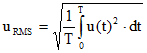Where, T is the time period of the signal Now,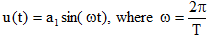Where, a1 is the peak value of the sinusoidal signal and ω is the angular frequency So,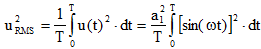Therefore,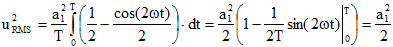So, the RMS value uRMS = a1/√2 = 0.707 times the peak value.

09․ To reduce hysteresis loss, we use
CRGO steel.
soft-iron.
laminated sheets.
copper bars.

Cold rolled grain oriented steel have grains aligned in the direction of rolling. When the magnetic flux passes through it, the magnetic domains get aligned in one direction causing minimum resistant path to flux causing lesser hysteresis loss.

10․ Unit of apparent power is
Watts.
VA.
VAR.
KWhr.

Apparent power is the product of voltage and current.

<<<4748495051>>>